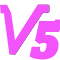$$\eta = \frac{E_{k}}{E_{k}+E_{R}} \quad (1)$$为了简化计算，我们考虑一种特殊情况：使用恒定磁场对弹丸进行加速。

$$E_{k}=Fx \quad (2)$$其中，F为线圈对弹丸产生的电磁力，x为加速距离。

$$E_{R} = I^{2}R_{D}t \quad (3)$$其中，I为线圈电流， $$R_{D}$$为驱动线圈电阻，t为加速时间。

$$E_{R} = I^{2}R_{D}\sqrt{\frac{2mx}{F}} \quad (4)$$所以效率η 可以表示为
$$\eta = \frac{1}{ 1 + \frac{ I^{2}R_{D} \sqrt{2m} } { F\sqrt{Fx} } } \quad (5)$$

$$F=kI$$ 也可以表示为 $$I=\frac{F}{k}$$将其代入式（5），可以得到
$$\eta = \frac{1}{ 1 + \frac{ R_{D} }{ k^{2} } \sqrt{ \frac{ 2Fm }{ x } } } \quad (6)$$这里，加速力F可以用速度v，初速度$$v_{0}$$，加速距离x，弹丸质量m表示。即
$$\eta = \frac{1}{ 1 + \frac{ mR_{D} }{ k^{2}x } \sqrt{v^{2}-{v_{0}}^{2}} } \quad (7)$$当初速度为0时，上式简化为
$$\eta = \frac{1}{ 1 + \frac{ mvR_{D} }{ k^{2}x } } \quad (8)$$式中，m为弹丸质量；v为弹丸出速度；$$R_{D}$$为线圈电阻；k为一比例系数，表示产生线圈对弹丸加速力与线圈电流的比值；x为加速距离。

[修改于 4年8个月前 - 2017/12/09 18:13:21]

+1  学术分虎哥    2016/11/16 长期从事电磁枪理论推导，为相关爱好者提供基础研制依据。

55年9个月前 修改于 5年9个月前

##### 200字以内，仅用于支线交流，主线讨论请采用回复功能。5年9个月前

“最优化的磁阻式，效率大于等于此值”希望讲解一下为什么不是小于计算值。

##### 200字以内，仅用于支线交流，主线讨论请采用回复功能。5年9个月前 修改于 5年9个月前

“最优化的磁阻……

（第一个学术分，开心\(^o^)/ ）

##### 200字以内，仅用于支线交流，主线讨论请采用回复功能。rb-sama5年9个月前

ー> I*m*V*Rd/k

m也是如此，则能量难保证。v 也是如此。

##### 200字以内，仅用于支线交流，主线讨论请采用回复功能。5年9个月前

##### 200字以内，仅用于支线交流，主线讨论请采用回复功能。进士 专家 学者 机友 笔友

54

970

4
2014/04/30注册，5时59分前活动

{{errorInfo}}

##### 当前账号的附件下载数量限制如下：

{{f.startingTime}}点 - {{f.endTime}}点 {{f.fileCount}}

{{tip}}
{{reason.type}}

#### 空空如也

{{format('YYYY/MM/DD HH:mm:ss', toc)}}